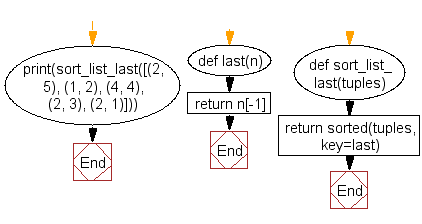﻿ Python: Get a list, sorted in increasing order by the last element in each tuple from a given list of non-empty tuples - w3resource# Python: Get a list, sorted in increasing order by the last element in each tuple from a given list of non-empty tuples

## Python List: Exercise-6 with Solution

Write a Python program to get a list, sorted in increasing order by the last element in each tuple from a given list of non-empty tuples.

Sample Solution:-

Python Code:

``````def last(n): return n[-1]

def sort_list_last(tuples):
return sorted(tuples, key=last)

print(sort_list_last([(2, 5), (1, 2), (4, 4), (2, 3), (2, 1)]))
```
```

Sample Output:

```[(2, 1), (1, 2), (2, 3), (4, 4), (2, 5)]
```

Pictorial Presentation:Flowchart:## Visualize Python code execution:

The following tool visualize what the computer is doing step-by-step as it executes the said program:

Python Code Editor:

Have another way to solve this solution? Contribute your code (and comments) through Disqus.

What is the difficulty level of this exercise?

Test your Python skills with w3resource's quiz

﻿

## Python: Tips of the Day

Floor Division:

When we speak of division we normally mean (/) float division operator, this will give a precise result in float format with decimals.

For a rounded integer result there is (//) floor division operator in Python. Floor division will only give integer results that are round numbers.

```print(1000 // 300)
print(1000 / 300)```

Output:

```3
3.3333333333333335```